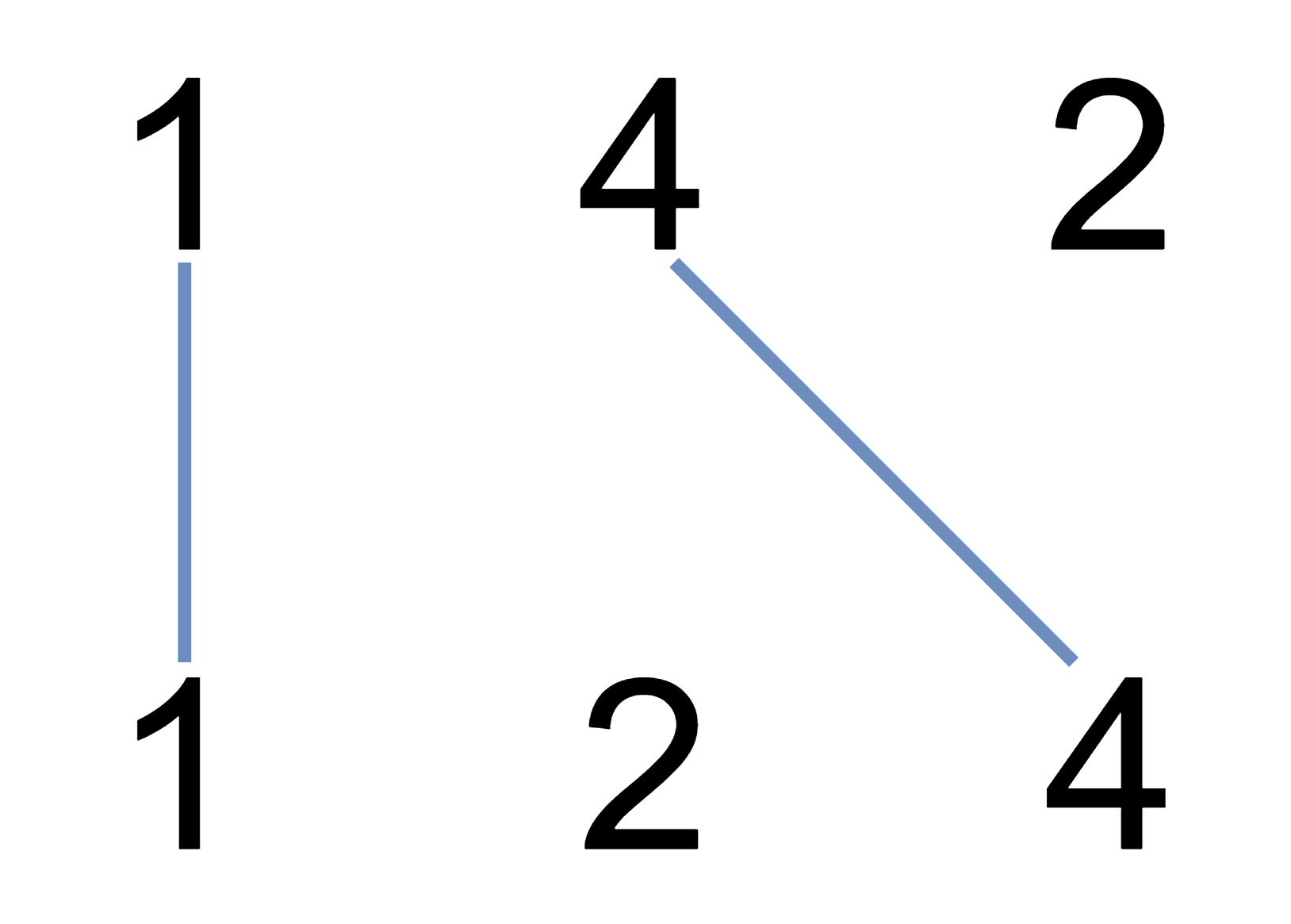578. Uncrossed Lines

0

Medium

You are given two integer arrays nums1 and nums2. We write the integers of nums1 and nums2 (in the order they are given) on two separate horizontal lines. We may draw connecting lines: a straight line connecting two numbers nums1[i] and nums2[j] such that: nums1[i] == nums2[j], and
the line we draw does not intersect any other connecting (non-horizontal) line.
Note that a connecting line cannot intersect even at the endpoints (i.e., each number can only belong to one connecting line). Print the maximum number of connecting lines we can draw in this way.Input Format

First Line contains size of first array.
Second Line contains element of first array.
Third Line contains size of second array.
Fourth Line contains element of second array.

Output Format

Print the maximum number of connecting lines we can draw in this way.(Integer)

Example

Input

3 1 4 2 3 1 2 4

Output

2

Constraints

1 <= nums1.length, nums2.length <= 500
1 <= nums1[i], nums2[j] <= 2000